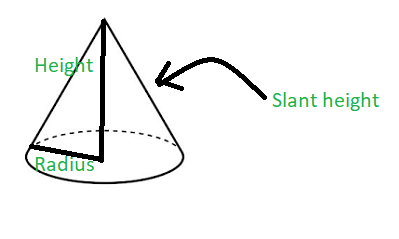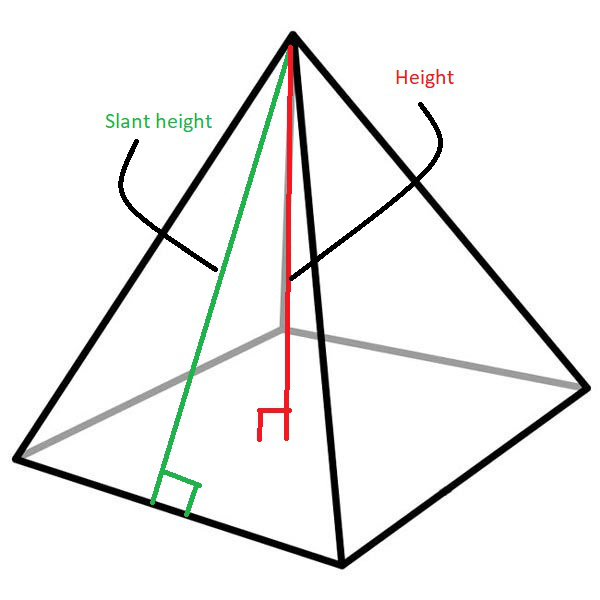# Program to find slant height of cone and pyramid

• Difficulty Level : Easy
• Last Updated : 07 Aug, 2022

Given two integers H1 and R representing the height and radius of a cone and two integers H2 and S representing the height and length of base of a pyramid, the task is to find the slant height of the cone and the pyramid.

Examples:

Input: H1 = 4.5, R = 6, H2 = 4, S = 4.8
Output:
Slant height of cone is: 7.5
Slant height of pyramid is: 4.66476

Input: H1 = 2, R = 4, H2 = 4, S = 8
Output:
Slant height of cone is: 4.47214
Slant height of pyramid is: 5.65685

Approach: The slant height of an object such as a cone or a pyramid is the distance measured from any vertex along a lateral face to the base (along the center of the face). The slant height of a right circular cone is uniform throughout the surface and is given by the formula:where,
L is the slant height of the right circular cone
R is the radius of the right circular cone and
H is the height of the right circular cone

The slant height of a pyramid is given by the formula:where,
L is the slant height of the pyramid
S is the side of the base of the pyramid
H is the height of the pyramid

Below is the implementation of the above approach:

## C++

 // C++ program for the above approach #include  using namespace std;   // Function to calculate slant // height of a cone void coneSlantHeight(double cone_h,                      double cone_r) {     // Store the slant height of cone     double slant_height_cone         = sqrt(pow(cone_h, 2)                + pow(cone_r, 2));       // Print the result     cout << "Slant height of cone is: "          << slant_height_cone << '\n'; }   // Function to find the slant // height of a pyramid void pyramidSlantHeight(double pyramid_h,                         double pyramid_s) {       // Store the slant height of pyramid     double slant_height_pyramid         = sqrt(pow(pyramid_s / 2, 2)                + pow(pyramid_h, 2));       // Print the result     cout << "Slant height of pyramid is: "          << slant_height_pyramid << '\n'; }   // Driver Code int main() {     // Dimensions of Cone     double H1 = 4.5, R = 6;       // Function Call for slant height     // of Cone     coneSlantHeight(H1, R);       // Dimensions of Pyramid     double H2 = 4, S = 4.8;       // Function to calculate     // slant height of a pyramid     pyramidSlantHeight(H2, S);       return 0; }

## Java

 // Java program for the above approach import java.io.*; class GFG  {           // Function to calculate slant     // height of a cone     static void coneSlantHeight(double cone_h,                          double cone_r)     {                 // Store the slant height of cone         double slant_height_cone             = Math.sqrt(Math.pow(cone_h, 2)                    + Math.pow(cone_r, 2));               // Print the result         System.out.println("Slant height of cone is: " +          slant_height_cone);     }           // Function to find the slant     // height of a pyramid     static void pyramidSlantHeight(double pyramid_h,                             double pyramid_s)     {               // Store the slant height of pyramid         double slant_height_pyramid             = Math.sqrt(Math.pow(pyramid_s / 2, 2)                    + Math.pow(pyramid_h, 2));               // Print the result         System.out.println("Slant height of pyramid is: " +          slant_height_pyramid);     }           // Driver Code     public static void main (String[] args)     {                 // Dimensions of Cone         double H1 = 4.5, R = 6;               // Function Call for slant height         // of Cone         coneSlantHeight(H1, R);               // Dimensions of Pyramid         double H2 = 4, S = 4.8;               // Function to calculate         // slant height of a pyramid         pyramidSlantHeight(H2, S);           } }   // This code is contributed by AnkThon

## Python3

 # Python 3 program for the above approach from math import sqrt,pow # Function to calculate slant # height of a cone def coneSlantHeight(cone_h, cone_r):   # Store the slant height of cone   slant_height_cone = sqrt(pow(cone_h, 2) + pow(cone_r, 2))     # Print the result   print("Slant height of cone is:",slant_height_cone)   # Function to find the slant # height of a pyramid def pyramidSlantHeight(pyramid_h, pyramid_s):   # Store the slant height of pyramid   slant_height_pyramid = sqrt(pow(pyramid_s/2, 2) + pow(pyramid_h, 2))      # Print the result   print("Slant height of pyramid is:","{:.5f}".format(slant_height_pyramid))   # Driver Code if __name__ == '__main__':   # Dimensions of Cone   H1 = 4.5   R = 6     # Function Call for slant height   # of Cone   coneSlantHeight(H1, R);     # Dimensions of Pyramid   H2 = 4   S = 4.8     # Function to calculate   # slant height of a pyramid   pyramidSlantHeight(H2, S)

## C#

 // C# program for the above approach using System; public class GFG  {     // Function to calculate slant   // height of a cone   static void coneSlantHeight(double cone_h,                               double cone_r)   {       // Store the slant height of cone     double slant_height_cone       = Math.Sqrt(Math.Pow(cone_h, 2)                   + Math.Pow(cone_r, 2));       // Print the result     Console.WriteLine("Slant height of cone is: " +                        slant_height_cone);   }     // Function to find the slant   // height of a pyramid   static void pyramidSlantHeight(double pyramid_h,                                  double pyramid_s)   {       // Store the slant height of pyramid     double slant_height_pyramid       = Math.Sqrt(Math.Pow(pyramid_s / 2, 2)                   + Math.Pow(pyramid_h, 2));       // Print the result     Console.WriteLine("Slant height of pyramid is: " +                        slant_height_pyramid);   }     // Driver Code   public static void Main (string[] args)   {       // Dimensions of Cone     double H1 = 4.5, R = 6;       // Function Call for slant height     // of Cone     coneSlantHeight(H1, R);       // Dimensions of Pyramid     double H2 = 4, S = 4.8;       // Function to calculate     // slant height of a pyramid     pyramidSlantHeight(H2, S);     } }   // This code is contributed by AnkThon

## Javascript

 

Output:

Slant height of cone is: 7.5
Slant height of pyramid is: 4.66476

Time Complexity: O(sqrt(logH1+logR) + sqrt(logH2+logS))
Auxiliary Space: O(1)

My Personal Notes arrow_drop_up
Recommended Articles
Page :